# Offline SSVEP-based BCI Multiclass Prediction¶

Building extended covariance matrices for SSVEP-based BCI. The obtained matrices are shown. A Minimum Distance to Mean classifier is trained to predict a 4-class problem for an offline setup.

# Authors: Sylvain Chevallier <sylvain.chevallier@uvsq.fr>,
# Emmanuel Kalunga, Quentin Barthélemy, David Ojeda
#

import numpy as np
import matplotlib.pyplot as plt

from mne import find_events, Epochs
from mne.io import Raw
from sklearn.model_selection import cross_val_score, RepeatedKFold

from pyriemann.estimation import BlockCovariances
from pyriemann.utils.mean import mean_riemann
from pyriemann.classification import MDM


# Download data
# Read data in MNE Raw and numpy format
events = find_events(raw, shortest_event=0, verbose=False)
raw = raw.pick_types(eeg=True)

event_id = {'13 Hz': 2, '17 Hz': 4, '21 Hz': 3, 'resting-state': 1}
sfreq = int(raw.info['sfreq'])
eeg_data = raw.get_data()

Using default location ~/mne_data for ssvep...

0%|                                              | 0.00/3.33M [00:00<?, ?B/s]
1%|▎                                     | 31.7k/3.33M [00:00<00:14, 228kB/s]
2%|▋                                     | 64.5k/3.33M [00:00<00:14, 231kB/s]
3%|█                                     | 97.3k/3.33M [00:00<00:13, 232kB/s]
5%|██                                     | 179k/3.33M [00:00<00:08, 371kB/s]
12%|████▌                                  | 392k/3.33M [00:00<00:03, 785kB/s]
24%|█████████▏                            | 802k/3.33M [00:00<00:01, 1.51MB/s]
49%|██████████████████                   | 1.62M/3.33M [00:00<00:00, 2.93MB/s]
85%|███████████████████████████████▍     | 2.83M/3.33M [00:01<00:00, 4.61MB/s]
99%|████████████████████████████████████▌| 3.29M/3.33M [00:01<00:00, 3.25MB/s]
0%|                                              | 0.00/3.33M [00:00<?, ?B/s]
100%|█████████████████████████████████████| 3.33M/3.33M [00:00<00:00, 13.7GB/s]
/home/docs/checkouts/readthedocs.org/user_builds/pyriemann/checkouts/latest/examples/SSVEP/helpers/ssvep_helpers.py:79: RuntimeWarning: Setting non-standard config type: "MNE_DATASETS_SSVEPEXO_PATH"
data_path = fetch_dataset(dataset_params, force_update=True)


## Visualization of raw EEG data¶

Plot few seconds of signal from the Oz electrode using matplotlib

n_seconds = 2
time = np.linspace(0, n_seconds, n_seconds * sfreq,
endpoint=False)[np.newaxis, :]
plt.figure(figsize=(10, 4))
plt.plot(time.T, eeg_data[np.array(raw.ch_names) == 'Oz', :n_seconds*sfreq].T,
color='C0', lw=0.5)
plt.xlabel("Time (s)")
plt.ylabel(r"Oz ($\mu$V)")
plt.show()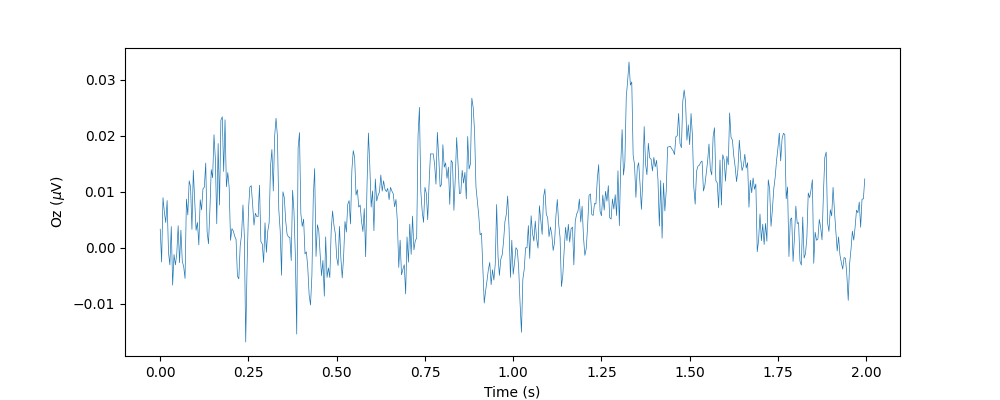And of all electrodes:

plt.figure(figsize=(10, 4))
for ch_idx, ch_name in enumerate(raw.ch_names):
plt.plot(time.T, eeg_data[ch_idx, :n_seconds*sfreq].T, lw=0.5,
label=ch_name)
plt.xlabel("Time (s)")
plt.ylabel(r"EEG ($\mu$V)")
plt.legend(loc='upper right')
plt.show()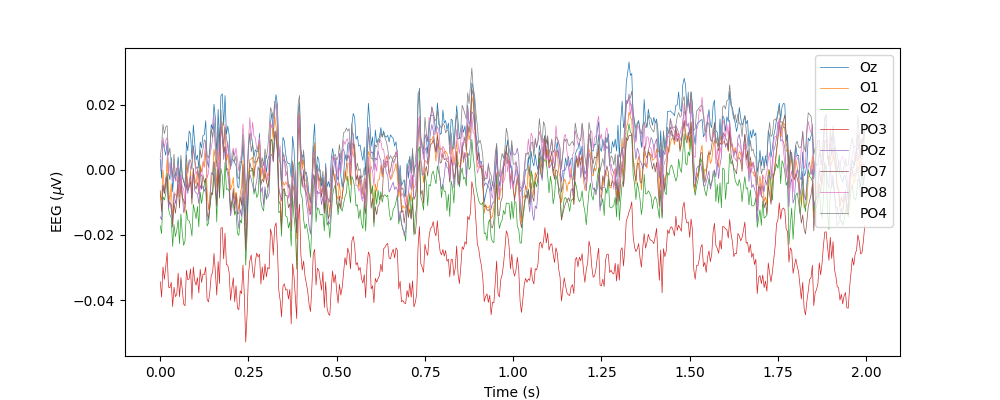With MNE, it is much easier to visualize the data

raw.plot(duration=n_seconds, start=0, n_channels=8, scalings={'eeg': 4e-2},
color={'eeg': 'steelblue'})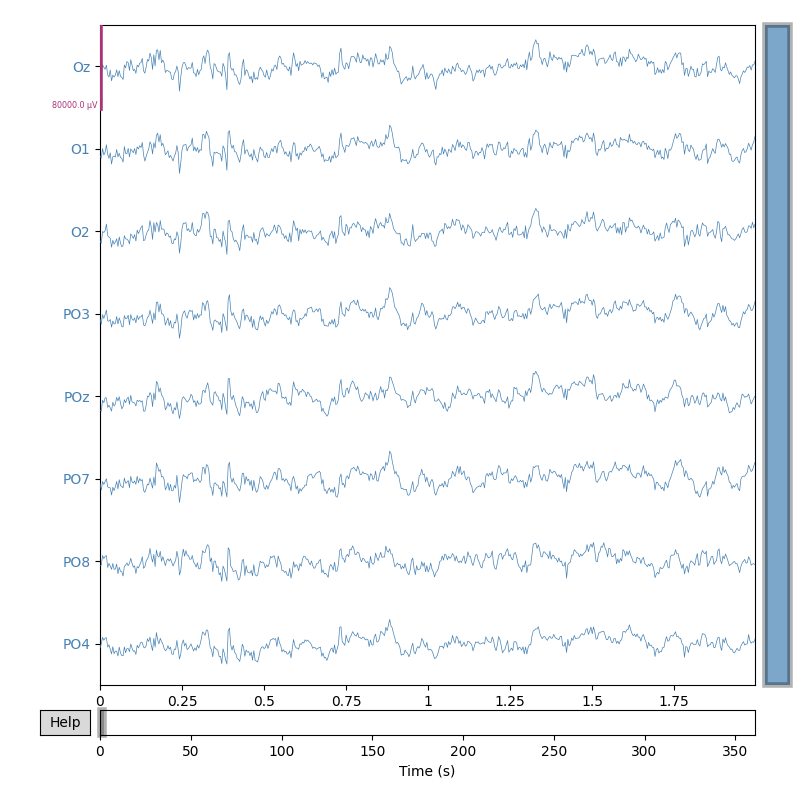Using matplotlib as 2D backend.

<MNEBrowseFigure size 800x800 with 4 Axes>


## Extended signals for spatial covariance¶

Using the approach proposed by 1, the SSVEP signal is extended to include the filtered signals for each stimulation frequency. We stack the filtered signals to build an extended signal.

# We stack the filtered signals to build an extended signal
frequencies = [13, 17, 21]
freq_band = 0.1
raw_ext = extend_signal(raw, frequencies, freq_band)

Creating RawArray with float64 data, n_channels=24, n_times=92384
Range : 0 ... 92383 =      0.000 ...   360.871 secs


Plot the extended signal

raw_ext.plot(duration=n_seconds, start=14, n_channels=24,
scalings={'eeg': 5e-4}, color={'eeg': 'steelblue'})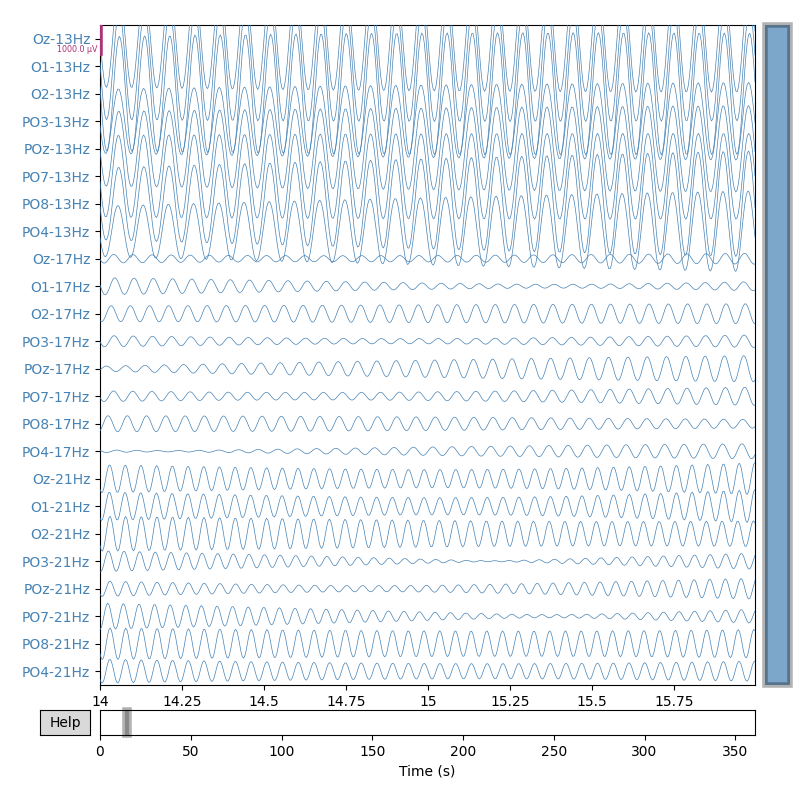<MNEBrowseFigure size 800x800 with 4 Axes>


Building Epochs and plotting 3 s of the signal from electrode Oz for a trial

epochs = Epochs(raw_ext, events, event_id, tmin=2, tmax=5, baseline=None)

n_seconds = 3
time = np.linspace(0, n_seconds, n_seconds * sfreq,
endpoint=False)[np.newaxis, :]
channels = range(0, len(raw_ext.ch_names), len(raw.ch_names))
plt.figure(figsize=(7, 5))
for f, c in zip(frequencies, channels):
plt.plot(epochs.get_data()[5, c, :].T, label=str(int(f))+' Hz')
plt.xlabel("Time (s)")
plt.ylabel(r"Oz after filtering ($\mu$V)")
plt.legend(loc='upper right')
plt.show()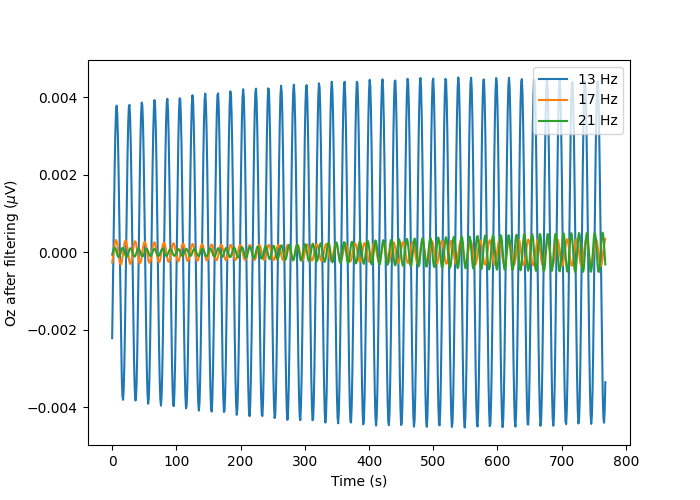Not setting metadata
32 matching events found
No baseline correction applied
0 projection items activated
Using data from preloaded Raw for 32 events and 769 original time points ...
Using data from preloaded Raw for 32 events and 769 original time points ...
Using data from preloaded Raw for 32 events and 769 original time points ...


As it can be seen on this example, the subject is watching the 13Hz stimulation and the EEG activity is showing an increase activity in this frequency band while other frequencies have lower amplitudes.

## Spatial covariance for SSVEP¶

The covariance matrices will be estimated using the Ledoit-Wolf shrinkage estimator on the extended signal.

cov_ext_trials = BlockCovariances(estimator='lwf',
block_size=8).transform(epochs.get_data())

# This plot shows an example of a covariance matrix observed for each class:
ch_names = raw_ext.info['ch_names']

plt.figure(figsize=(7, 7))
for i, l in enumerate(event_id):
ax = plt.subplot(2, 2, i+1)
plt.imshow(cov_ext_trials[events[:, 2] == event_id[l]],
cmap=plt.get_cmap('RdBu_r'))
plt.title('Cov for class: '+l)
plt.xticks([])
if i == 0 or i == 2:
plt.yticks(np.arange(len(ch_names)), ch_names)
ax.tick_params(axis='both', which='major', labelsize=7)
else:
plt.yticks([])
plt.show()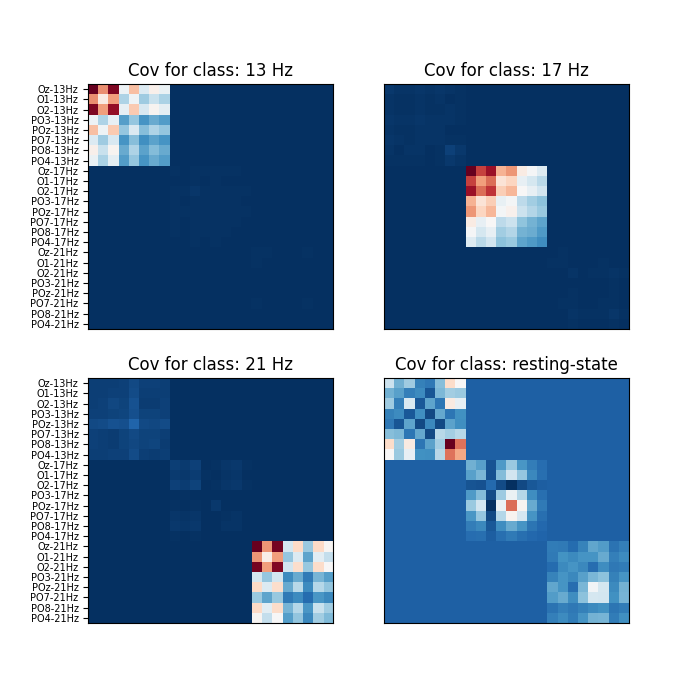Using data from preloaded Raw for 32 events and 769 original time points ...


It appears clearly that each class yields a different structure of the covariance matrix. Each stimulation (13, 17 and 21 Hz) generating higher covariance values for EEG signal filtered at the proper bandwith and no activation at all for the other bandwiths. The resting state, where the subject focus on the center of the display and far from all blinking stimulus, shows an activity with higher correlation in the 13Hz frequency and lower but still visible activity in the other bandwiths.

## Classify with MDM¶

Plotting mean of each class

cov_centers = np.empty((len(event_id), 24, 24))
for i, l in enumerate(event_id):
cov_centers[i] = mean_riemann(cov_ext_trials[events[:, 2] == event_id[l]])

plt.figure(figsize=(7, 7))
for i, l in enumerate(event_id):
ax = plt.subplot(2, 2, i+1)
plt.imshow(cov_centers[i], cmap=plt.get_cmap('RdBu_r'))
plt.title('Cov mean for class: '+l)
plt.xticks([])
if i == 0 or i == 2:
plt.yticks(np.arange(len(ch_names)), ch_names)
ax.tick_params(axis='both', which='major', labelsize=7)
else:
plt.yticks([])
plt.show()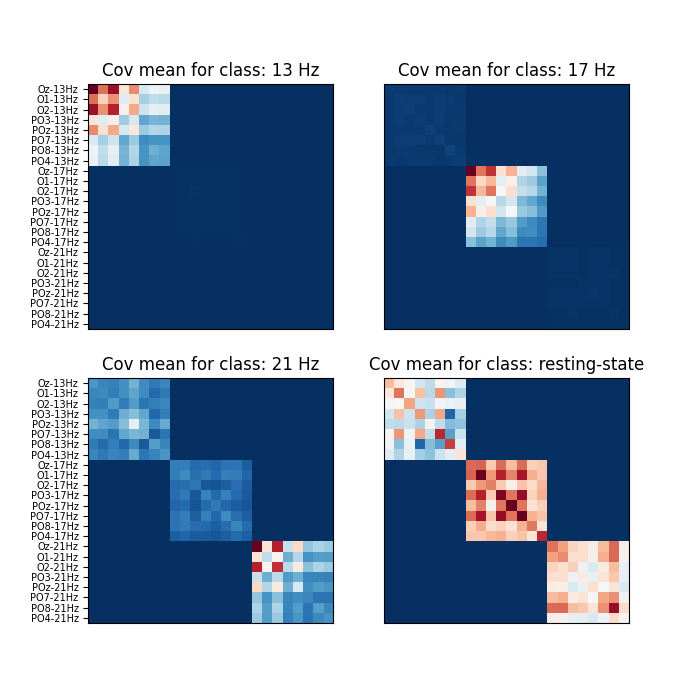Minimum distance to mean is a simple and robust algorithm for BCI decoding. It reproduces results of 2 for the first session of subject 12.

print("Number of trials: {}".format(len(cov_ext_trials)))

cv = RepeatedKFold(n_splits=2, n_repeats=10, random_state=42)
mdm = MDM(metric=dict(mean='riemann', distance='riemann'))
scores = cross_val_score(mdm, cov_ext_trials, events[:, 2], cv=cv, n_jobs=1)
print("MDM accuracy: {:.2f}% +/- {:.2f}".format(np.mean(scores)*100,
np.std(scores)*100))
# The obtained results are 80.62% +/- 16.29 for this session, with a repeated
# 10-fold validation.

Number of trials: 32
warnings.warn('Convergence not reached')
warnings.warn('Convergence not reached')
warnings.warn('Convergence not reached')
warnings.warn('Convergence not reached')
warnings.warn('Convergence not reached')
warnings.warn('Convergence not reached')
warnings.warn('Convergence not reached')
warnings.warn('Convergence not reached')